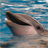# The computing power of the Vivante's GC2000 GPU in i.mx6q (more)

cancel
Showing results for
Did you mean:

## The computing power of the Vivante's GC2000 GPU in i.mx6q (more)

9,598 ViewsContributor III

Here is the real power of Vivante's GC2000 GPU in i.MX6 Q
4 Computing power tests
The matrix multiplication algorithm was used to test it power. For comparison, a Lenovo Thinkpad x200 was used, it has 64-bit Ubuntu 12.04 and Intel OpenCL driver installed, 4G memory, Core 2 Duo CPU P8600 @ 2.40GHz × 2.

4.1 Theoretical Power
Let's first figure out how fast the GC2000 can compute theoretically.
GC2000 has four compute units, each unit can handle four float MAD (multiply and add) operation, if every unit of GC2000 can make a MAD operation in every clock cycle, and the GPU's maximum frequency is 500MHZ, so GC2000 would have a computing power : 0.5*4*4*2 = 16GFLOPS.
We first make a kernel program with no meaningful operations, just computation
between registers of GPU. Here is one:
"__kernel void vadd (__global const float4 *a, __global const float4 *b, __global float4 *c){ \n",
" int gid = get_global_id(0); \n",
" int i; \n",
" float4 dd1, dd2, dd3, dd4, dd5; \n",
" for(i=0; i<900000; i++) { \n",
"dd3 += dd2*dd5; \n",
"dd2 += dd1*dd4; \n",
"dd4 += dd3*dd1; \n",
"dd1 += dd3*dd2; \n",
"dd3 += dd2*dd5; \n",
"dd2 += dd1*dd4; \n",
"dd4 += dd3*dd1; \n",
"dd1 += dd3*dd2; \n",
"}\n"
"}\n"
The result is 13.8GFLOPS, that is a rather reasonable figure.

4.2 Real power
We used the GPU to compute the multiplication of two 1000x1000 matrix. The total computation load is 2*1000^3=2GFLOPS.
Here is the kernel program:
"__kernel void mmul(const int Ndim, const int Pdim, const int Mdim, __global float4 *A, __global float4 *B, __global float *C) { \n",

"int k, kk, m1, m2; \n",
"int i = get_global_id(0); \n",
"int j = get_global_id(1); \n",
"float4 tmp=0.0; \n",
"m1 = i*(Pdim/8); m2=j*(Pdim/8); \n",
"for(k=0; k<(Pdim/8); k++, m1++, m2++) \n",
"tmp += Atmp1[kk] * tmp2[kk];\n",
"C[i*Mdim+j] = tmp.x+tmp.y+tmp.z+tmp.w; \n",
"}\n"
The program used 3.3 seconds, that is about 600MFLOPS, or 0.6GFLOPS.
For comparison, the same program ran on the X200 laptop with Intel's OpenCL driver,  the result is quite surprising: 2.9GFLOPS.

Let's figure out the reason for the difference:
First, The Cuo 2 P8600 has two CPU cores, the working frequency is 2.4G, each core has a SSE 128bit floating point processor, it can be regarded as one shader, so P8600 has two shaders working at 2.4G, GC2000 has 4 shaders working at 0.5G, the ratio of computing ability shall be 2*2.4 : 4*0.5 = 2.4 : 1.
Secondly, P8600 has 3M L2 cache, and for GC2000, as stated above, the OpenCL can only  use 1k local memory and 64byte memory cache.
And further, the memory bandwidth greatly influenced the result. Intel's P8600 memory bandwith is 800Mhz 64bit, that is 6.4G. According to I.MX6's datasheet, the double 64bit AXI can run at maximum speed of 264Mhz, the bandwith is about 2.1G. As GC2000 almost has no cache, one GLOPS needs at least one new float number, that is 4 bytes, for a 2.1G bus, the maximum throughput is 500M float numbers, cache can make things a little better, so the test result 600MGLOPS is a very reasonable figure for GC2000.

600MFLOS is not a very bad result. Half of it is enough to drive a 720p display. So, there is plenty of room for general computing.

As you see, the bottleneck of GPU computing is the system bus bandwidth and cache size of the GPU. I do not know why Vivante designed such a small cache for OpenCL. Maybe it is the driver's configuration, or the hardware's limitation. That tremendously limited the power of gc2000.
I hope the engineers of Vivante could make some optimization for their driver and future GPU design, so that their GPU could be better used for general computing.

The test platform is a i.MX6Q TV stick Hi802 with Ubuntu 11.10 linaro and  vivance opencl driver , the test platform for Lenovo X200 Laptop is Ubuntu 12.04 with Intel OpenCL driver.

Labels (1)

Tags (3)
2 Replies
891 ViewsContributor V

i have a gk802 (same as hi802). would you be able to provide your source code such that I could run this on my setup as well? thanks

891 ViewsContributor III

the code is there. with a trivial opencl main function, you will get it work. But first you must get the Ubuntu work on gk802, and get the opencl driver work.